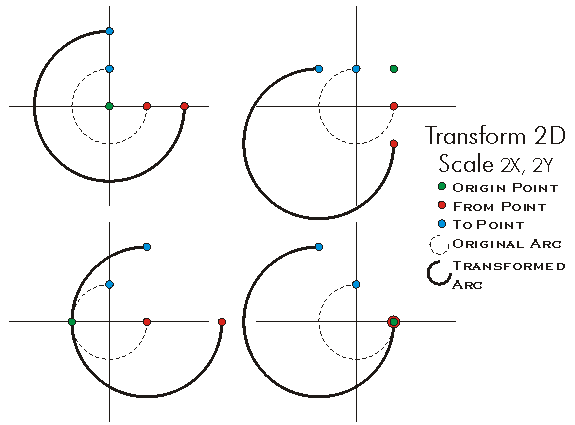This document is archived and information here might be outdated.  Recommended version.

ITransform2D.Scale Method (ArcObjects .NET 10.8 SDK)
 ArcObjects Help for .NET developers > ArcObjects Help for .NET developers > ArcObjects namespaces > Geometry > ESRI.ArcGIS.Geometry > Interfaces > IT > ITransform2D Interface > ITransform2D.Scale Method
 ArcGIS Developer Help

# ITransform2D.Scale Method

Scales about the specified origin using seperate horizonal and vertical scales. The origin point can be in a different spatial reference than the geometry being scaled.

```[Visual Basic .NET]
Public Sub Scale ( _
ByVal Origin As IPoint, _
ByVal sx As Double, _
ByVal sy As Double _
)
```
```[C#]
public void Scale (
IPoint Origin,
double sx,
double sy
);
```
```[C++]
HRESULT Scale(
IPoint* Origin,
double sx,
double sy
);
```
```[C++]
Parameters
Origin
Origin is a parameter of type IPoint*
sx
sx is a parameter of type double
sy
sy is a parameter of type double
```

#### Product Availability

Available with ArcGIS Engine, ArcGIS Desktop, and ArcGIS Server.

#### Description

Stretches the Geometry a factor of sX along the X-Axis and a factor of sY along the Y-Axis (where sX is the ratio of Old Width to New Width, and sY is the ratio of Old Height to New Height).  The Origin point is the reference Point from which the transformation is performed (Regardless of the location of the Origin point, the Geometry resulting from the transformation is the same, except for a positional offset).  The Origin is the only point in the transformation guaranted to remain in the same location after the transformation is complete.

#### Remarks

Note: Caution must be taken when scaling a CircularArc or a geometry containing CircularArc segments.  Unless Abs(ScaleX) = Abs(ScaleY), the resulting CircularArcs will not retain the characteristics of the original geometry (since they remain CircularArcs).[C#]

//Create Point you wish to scale
IPoint startingPoint = new PointClass() as IPoint;
startingPoint.PutCoords(10, 10);
//Create a point to act as origin
IPoint origin = new PointClass() as IPoint;
origin.PutCoords(15, 15);
//Cast the startingPoint into the correct Interface
ITransform2D transformScalePoint = startingPoint as ITransform2D;
//Perform Scale
transformScalePoint.Scale(origin, .5, .5);
double x;
double y;
startingPoint.QueryCoords(out x, out y);
Debug.Print(x.ToString());
Debug.Print(y.ToString());